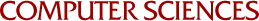## On the Computation of Rigorous Bounds for the Solutions of Linear Integral Equations With the Aid of Interval Arithmetic

C.W. Cryer
1969

A method is given for approximately solving linear Fredholm integral equations of the second klnd with non-negative kernels. The basis of the method is the construction of piecewise-polynomial degenerate kernels which bound the given kernel. The method is a generalization of a method suggested by Gerberich. When implemented on a computer, interval arithmetic is used so that rigorous bounds for the solution of the integral equations are obtained. The method is applied to two problems: the equation considered by Gerberich; and the equation of Love which arises in connection with the problem of determining the capacity of a circular plate condenser.